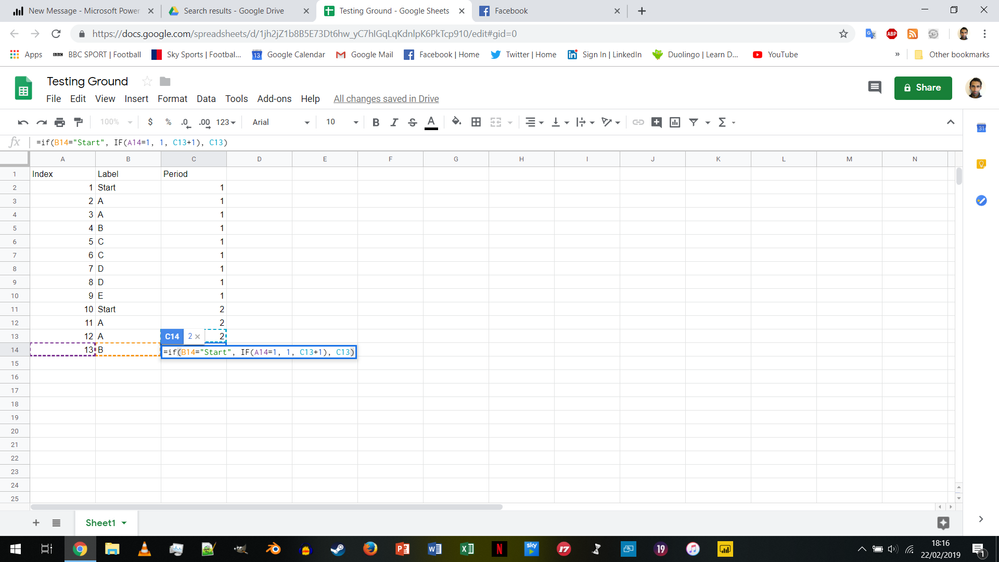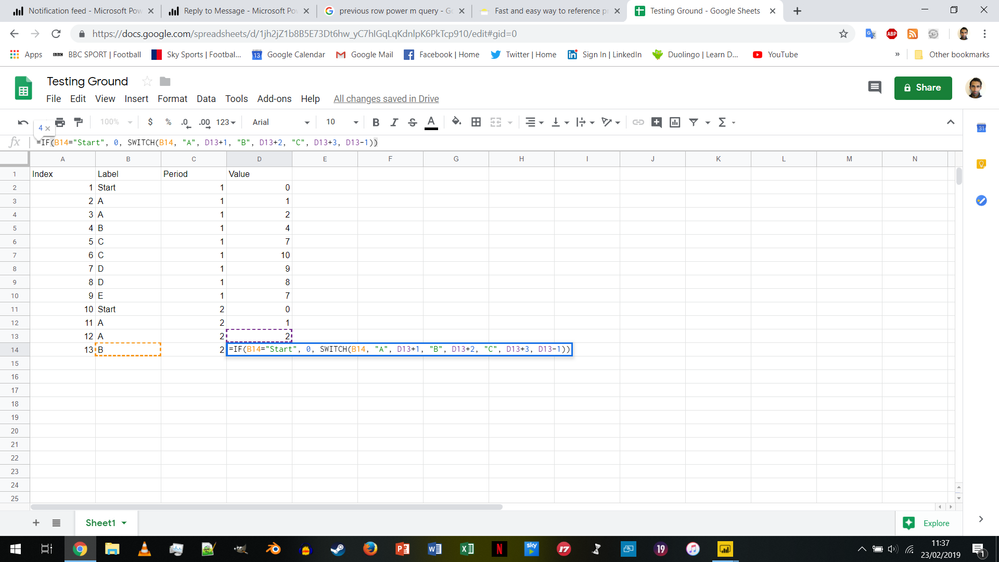cancel
Showing results for
Did you mean:Helper I

## Calculated column with previous row referencing same calculated column (DAX or Power M)

Hi,

I'm trying to create a new column in my table that relies on data from another column in the same row and a previous row from the row I'm trying to calculate.

My understanding is that DAX can't do it (from everything I've read you can't reference a previous row in the column you're trying to calculate - although very happy to be corrected on this), but that Power M might be able to via List. Accumulate?

I have almost no experience with Power M so any help would be helpful. I've posted an image of what I'm trying to achieve below.2 ACCEPTED SOLUTIONSSuper User

HI @xarius ,

You need to do the following:

• Filter out all the rows with Start
• Add a new index column starting in 1
• Merge the last step before the filter with the new step
• Do a fill down on the period column.

Check M code below and attach PBIX:

```let
Source = Table.FromRows(Json.Document(Binary.Decompress(Binary.FromText("i45WCi5JLCpRitWJVnJEIp3ApDMS6YJEuoJJnDpjAQ==", BinaryEncoding.Base64), Compression.Deflate)), let _t = ((type text) meta [Serialized.Text = true]) in type table [Label = _t]),
#"Changed Type" = Table.TransformColumnTypes(Source,{{"Label", type text}}),
#"Filtered Rows" = Table.SelectRows(#"Added Index", each ([Label] = "Start")),
#"Filled Down" = Table.FillDown(#"Expanded Added Index",{"Period"})
in
#"Filled Down"```

Regards,

MFelix

Regards

Miguel Félix

Proud to be a Super User!

Check out my blog: Power BI em PortuguêsSuper User

Oh, sorry - just recognized the problem now.

Yes, you have to perform some recursice operation here:

```let
Source = Table.FromRows(Json.Document(Binary.Decompress(Binary.FromText("i45WMlTSUQouSSwqUYrViVYyAvIcwSxjOMsEyHICs0yBLGcwywzOMgeyXMAsCzjLEshyBbMMDVCMNzSEm2qIsMrQGGJDLAA=", BinaryEncoding.Base64), Compression.Deflate)), let _t = ((type text) meta [Serialized.Text = true]) in type table [Index = _t, Label = _t]),
#"Changed Type" = Table.TransformColumnTypes(Source,{{"Index", Int64.Type}, {"Label", type text}}),
GenerateList =
List.Skip(
List.Generate(
()=> [Result = 0, Counter = 0],
each [Counter] <= Table.RowCount(#"Changed Type"),
each [
Switch = [
Start = 0,
A = [Result] + 1,
B = [Result] + 2,
C = [Result] + 3
],
Result = try Record.Field(Switch, #"Changed Type"[Label]{[Counter]}) otherwise [Result]-1,
Counter = [Counter]+1
],
each [Result])
,1),
MergeColumns = Table.FromColumns(Table.ToColumns(Source) & {GenerateList}, Table.ColumnNames(Source) & {"ExpectedOutput"})
in
MergeColumns```

Imke Feldmann (The BIccountant)

If you liked my solution, please give it a thumbs up. And if I did answer your question, please mark this post as a solution. Thanks!

14 REPLIES 14Super User

HI @xarius ,

You need to do the following:

• Filter out all the rows with Start
• Add a new index column starting in 1
• Merge the last step before the filter with the new step
• Do a fill down on the period column.

Check M code below and attach PBIX:

```let
Source = Table.FromRows(Json.Document(Binary.Decompress(Binary.FromText("i45WCi5JLCpRitWJVnJEIp3ApDMS6YJEuoJJnDpjAQ==", BinaryEncoding.Base64), Compression.Deflate)), let _t = ((type text) meta [Serialized.Text = true]) in type table [Label = _t]),
#"Changed Type" = Table.TransformColumnTypes(Source,{{"Label", type text}}),
#"Filtered Rows" = Table.SelectRows(#"Added Index", each ([Label] = "Start")),
#"Filled Down" = Table.FillDown(#"Expanded Added Index",{"Period"})
in
#"Filled Down"```

Regards,

MFelix

Regards

Miguel Félix

Proud to be a Super User!

Check out my blog: Power BI em PortuguêsHelper I

Hi @MFelix,

Thank you for this, it will definitely be useful but I also maybe made my use case too simple as I was hoping to apply the same logic to other columns with a bit more of a complex calculation.

I still need to definitely reference a row before the one currently being calculated, please see my example below:Super User

Hi @ImkeF ,

Can you help out on this request?

Regards,

MFelix

Regards

Miguel Félix

Proud to be a Super User!

Check out my blog: Power BI em PortuguêsSuper User

I've written a function to fetch the previous row fast on large datasets here:

https://www.thebiccountant.com/2018/07/12/fast-and-easy-way-to-reference-previous-or-next-rows-in-po...

Pls let me know if you have problems implementing it.

Imke Feldmann (The BIccountant)

If you liked my solution, please give it a thumbs up. And if I did answer your question, please mark this post as a solution. Thanks!Helper I

Hi @ImkeF,

I've seen that post before too, but as far as I can tell you can only reference a previous row of a different column (one that already has data/is calculated), not the one that you are currently calculating? Is that correct?Super User

No, you can use it for your use case as well: By default, the function would return all columns of the previous row.

But as stated in sample 3, you can narrow it down to just one column you're interested in:Imke Feldmann (The BIccountant)

If you liked my solution, please give it a thumbs up. And if I did answer your question, please mark this post as a solution. Thanks!Helper I

But in your example the "Value" column is fully formed - I need to calculate the current row of "Value" based on the previous row of "Value" - please see my 2nd screenshot for an example of what I'm trying to achieve.Super User

Sorry, but I don't understand what you mean. Could you please post a link to a sample file so that I can show you how it works?

Thx

Imke Feldmann (The BIccountant)

If you liked my solution, please give it a thumbs up. And if I did answer your question, please mark this post as a solution. Thanks!Helper I

Here is a link to my sample file (for some reason I can't just upload a file):

Sample File

It contains 2 tables - the sample data table is what I have to work with, the final table is what I'm trying to achieve.

I think the screenshot below is the easiest to understand though for the column I'm trying to create though.Super User

Oh, sorry - just recognized the problem now.

Yes, you have to perform some recursice operation here:

```let
Source = Table.FromRows(Json.Document(Binary.Decompress(Binary.FromText("i45WMlTSUQouSSwqUYrViVYyAvIcwSxjOMsEyHICs0yBLGcwywzOMgeyXMAsCzjLEshyBbMMDVCMNzSEm2qIsMrQGGJDLAA=", BinaryEncoding.Base64), Compression.Deflate)), let _t = ((type text) meta [Serialized.Text = true]) in type table [Index = _t, Label = _t]),
#"Changed Type" = Table.TransformColumnTypes(Source,{{"Index", Int64.Type}, {"Label", type text}}),
GenerateList =
List.Skip(
List.Generate(
()=> [Result = 0, Counter = 0],
each [Counter] <= Table.RowCount(#"Changed Type"),
each [
Switch = [
Start = 0,
A = [Result] + 1,
B = [Result] + 2,
C = [Result] + 3
],
Result = try Record.Field(Switch, #"Changed Type"[Label]{[Counter]}) otherwise [Result]-1,
Counter = [Counter]+1
],
each [Result])
,1),
MergeColumns = Table.FromColumns(Table.ToColumns(Source) & {GenerateList}, Table.ColumnNames(Source) & {"ExpectedOutput"})
in
MergeColumns```

Imke Feldmann (The BIccountant)

If you liked my solution, please give it a thumbs up. And if I did answer your question, please mark this post as a solution. Thanks!Helper I

Perfect! Thank you!!Super User

You're welcome!

Just recognized that I missed a buffer for performance. For some reason, I cannot edit my answer above - so I will paste the new code here:

```let
Source = Table.FromRows(Json.Document(Binary.Decompress(Binary.FromText("i45WMlTSUQouSSwqUYrViVYyAvIcwSxjOMsEyHICs0yBLGcwywzOMgeyXMAsCzjLEshyBbMMDVCMNzSEm2qIsMrQGGJDLAA=", BinaryEncoding.Base64), Compression.Deflate)), let _t = ((type text) meta [Serialized.Text = true]) in type table [Index = _t, Label = _t]),
#"Changed Type" = Table.Buffer(Table.TransformColumnTypes(Source,{{"Index", Int64.Type}, {"Label", type text}})),
GenerateList =
List.Skip(
List.Generate(
()=> [Result = 0, Counter = 0],
each [Counter] <= Table.RowCount(#"Changed Type"),
each [
Switch = [
Start = 0,
A = [Result] + 1,
B = [Result] + 2,
C = [Result] + 3
],
Result = try Record.Field(Switch, #"Changed Type"[Label]{[Counter]}) otherwise [Result]-1,
Counter = [Counter]+1
],
each [Result])
,1),
MergeColumns = Table.FromColumns(Table.ToColumns(Source) & {GenerateList}, Table.ColumnNames(Source) & {"ExpectedOutput"})
in
MergeColumns```

Imke Feldmann (The BIccountant)

If you liked my solution, please give it a thumbs up. And if I did answer your question, please mark this post as a solution. Thanks!Super User

Hi @ImkeF ,

I have found that postnot really sure if could be use to implement in this case,  but was suspicious of that.

Regards,

MFelix

Regards

Miguel Félix

Proud to be a Super User!

Check out my blog: Power BI em PortuguêsHelper I

Just bumping this in the hope someone can help me with the second half of my problem! (@MFelix)